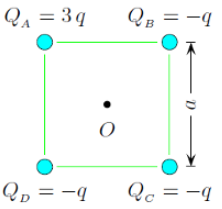# Problem: What is the magnitude of the electric field Ec at C due to the charges at A, B, and D?

###### FREE Expert Solution

Electric field,

$\overline{){\mathbf{E}}{\mathbf{=}}\frac{\mathbf{k}\mathbf{q}}{{\mathbf{r}}^{\mathbf{2}}}}$, where is Coulomb's constant, is the charge, and r is the distance from the point to the charge.

For the electric field at point C, we have:

$\overline{)\begin{array}{rcl}{\mathbf{E}}_{\mathbf{C}}& {\mathbf{=}}& \frac{\mathbf{k}{\mathbf{Q}}_{\mathbf{A}}}{{{\mathbf{r}}_{\mathbf{A}}}^{\mathbf{2}}}\mathbf{+}\frac{\mathbf{k}{\mathbf{Q}}_{\mathbf{B}}}{{{\mathbf{r}}_{\mathbf{B}}}^{\mathbf{2}}}\mathbf{+}\frac{\mathbf{k}{\mathbf{Q}}_{\mathbf{D}}}{{{\mathbf{r}}_{\mathbf{D}}}^{\mathbf{2}}}\end{array}}$

94% (481 ratings)###### Problem DetailsWhat is the magnitude of the electric field Ec at C due to the charges at A, B, and D?